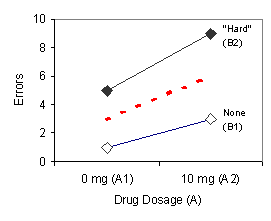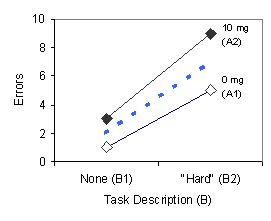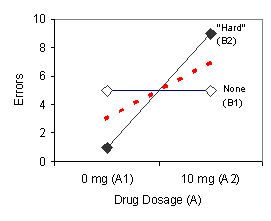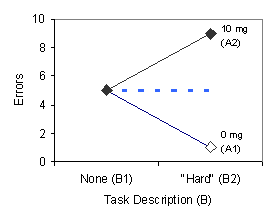## Factorial Designs: Main Effects

The main effect in a factorial design is "the effect of one independent variable averaged over all levels of another independent variable" (McBurney, 2004, p. 289). Table 4 below shows hypothetical data for our 2 x 2 factorial design example.

TABLE 4. Hypothetical data from a 2 x 2 factorial design with drug dosage as Factor A and task description as Factor B; this shows a main effect for both Factor A and Factor B.
None (B1) "Hard" (B2) Row Mean
DRUG DOSAGE (A) 0 mg (A1)
 1 5 3 9
3
10 mg (A2) 6
Column Mean 2 7

Figure 2 below shows how these main effects for Factor A and Factor B would look on a graph.FIGURE 2. Graphs illustrating a main effect for Factor A (left panel) and Factor B (right panel).

The two solid lines on the graph in the left panel show the effect of the drug (Factor A) for both levels of task description (Factor B). This graph illustrates mean number of errors as a function of drug dosage with task description as a parameter. Errors increase across the two levels of the drug (A1 and A2) both when subjects were told nothing about the task difficulty (line B1) and when they are told that the task was "hard" (line B2). The dashed red line shows the average increase (the two row means in Table 3) across the two levels of the drug; this is the main effect for Factor A. The two solid lines on the graph in the right panel show the effect of the task description (Factor B) for both levels of the drug (Factor A). This graph illustrates mean number of errors as a function of task description with drug dosage as a parameter. Errors increase across the two levels of task description (B1 and B2) when the pill does not contain the drug (line A1) and when the pill does contain the drug (line A2). The dashed blue line shows the average increase (the two column means in Table 2) across the two levels of task description; this is the main effect for Factor B.

What if there was no main effect for one or both of the factors? What might these data look like? Let's consider the case in which there is a main effect for Factor A and no main effect for Factor B. Such results are shown in Table 5 and the data are plotted in Figure 3, both presented below.

TABLE 5. Hypothetical data from a 2 x 2 factorial design with drug dosage as Factor A and task description as Factor B; this shows a main effect for Factor A only.